Courses

# Flywheel & Governor - MCQ Test 1

## 20 Questions MCQ Test GATE Mechanical (ME) 2022 Mock Test Series | Flywheel & Governor - MCQ Test 1

Description
This mock test of Flywheel & Governor - MCQ Test 1 for Mechanical Engineering helps you for every Mechanical Engineering entrance exam. This contains 20 Multiple Choice Questions for Mechanical Engineering Flywheel & Governor - MCQ Test 1 (mcq) to study with solutions a complete question bank. The solved questions answers in this Flywheel & Governor - MCQ Test 1 quiz give you a good mix of easy questions and tough questions. Mechanical Engineering students definitely take this Flywheel & Governor - MCQ Test 1 exercise for a better result in the exam. You can find other Flywheel & Governor - MCQ Test 1 extra questions, long questions & short questions for Mechanical Engineering on EduRev as well by searching above.
QUESTION: 1

### The ratio of maximum fluctuation of speed to the mean speed is called

Solution:

The co-efficient of fluctuation of speed is the ratio of maximum fluctuation of speed to the mean speed.

QUESTION: 2

Solution:
QUESTION: 3

### If Cf is the coefficient of speed fluctuation of a fly wheel then the ratio of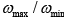will be

Solution: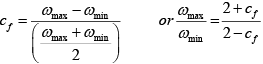QUESTION: 4

Given that m = mass of the ball of the governor,
ω = angular velocity of the governor and g = acceleration due to gravity,

The height of Watt's governor is given by

Solution:
QUESTION: 5

For a certain engine having an average speed of 1200 rpm, a flywheel approximated as a solid disc, is required for keeping the fluctuation of speed within 2% about the average speed. The fluctuation of kinetic energy per cycle is found to be 2 kJ. What is the least possible mass of the flywheel if its diameter is not to exceed 1m?

Solution: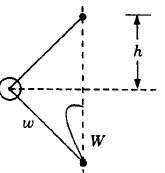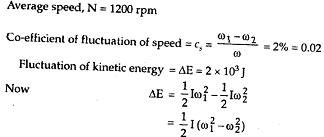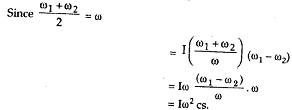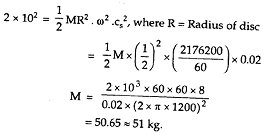QUESTION: 6

The speed of an engine varies from 210 rad/s to 190 rad/s. During the cycle the change in kinetic energy is found to be 400 Nm. The inertia of the flywheel in kg/m2 is

Solution:
QUESTION: 7

The speed of an engine varies from 210 rad/s to 190 rad/s. During a cycle the change in kinetic energy is found to be 400 Nm. The inertia of the flywheel in kgm2 is

Solution:
QUESTION: 8

Consider the following speed governors:

1. Porter governor
2. Hartnell governor
3. Watt governor
4. Proell Governor

The correct sequence of development of these governors is

Solution:

Watt,  Porter,  Proell, Hartnell.

QUESTION: 9

A fly wheel of moment of inertia 9.8 kgm2 fluctuates by 30 rpm for a fluctuation in energy of 1936 Joules. The mean speed of the flywheel is (in rpm)

Solution:
QUESTION: 10

Consider the given figure:Assertion (A): In order to have the same equilibrium speed for the given values of w, W and h, the masses of balls used in the Proell governor are less than those of balls used in the Porter governor.
Reason (R): The ball is fixed to an extension link in Proell governor.

Solution:
QUESTION: 11

Consider the following statements:

1. Flywheel and governor of an engine are the examples of an open loop control system
2. Governor is the example of closed loop control system
3. The thermostat of a refrigerator and relief valve of a boiler are the examples of closed loop control system

Which of these statements is/are correct?

Solution:
QUESTION: 12

In a Hartnell governor, the mass of each ball is 2.5 kg. Maximum and minimum speeds of rotation are 10 rad/s and 8 rad/s respectively. Maximum and minimum radii of rotation are 20 cm and 14 cm respectively. The lengths of horizontal and vertical arms of bell crank levers are 10 cm and 20 cm respectively. Neglecting obliquity and gravitational effects, the lift of the sleeve is

Solution:
QUESTION: 13

Which one of the following engines will have heavier flywheel than the remaining ones?

Solution:

The four stroke engine running at lower speed needs heavier fly wheel.

QUESTION: 14

For a given fractional change of speed, if the displacement of the sleeve is high, then the governor is said to be

Solution:
QUESTION: 15

A flywheel is fitted to the crankshaft of an engine having W as the amount of indicated work per revolution and permissible limits of coefficient of fluctuation of energy and speed as CEand CS respectively. The kinetic energy of the flywheel is given by

Solution:
QUESTION: 16

Which one of the following governors is used to drive a gramophone?

Solution:
QUESTION: 17

A rotating shaft carries a flywheel which overhangs on the bearing as a cantilever. If this flywheel weight is reduced to half of its original weight, the whirling speed will

Solution: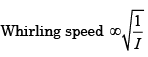QUESTION: 18

Which one of the following expresses the sensitiveness of a governor?

(Where N1 = Maximum equilibrium speed, N2 = Minimum equilibrium speed)

Solution:
QUESTION: 19

Assertion (A): In designing the size of the flywheel, the weight of the arms and the boss are neglected.
Reason (R): The flywheel absorbs energy during those periods when the turning moment is greater than the resisting moment.

Solution:
QUESTION: 20

Sensitiveness of a governor is defined as

Solution: